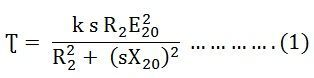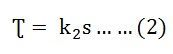# Torque Slip Characteristic of an Induction Motor

The Torque Slip Characteristic is represented by a rectangular hyperbola. For the immediate value of the slip, the graph changes from one form to the other. Thus, it passes through the point of maximum torque when R2 = sX20. The maximum torque developed in an induction motor is called the Pull Out Torque or the Breakdown Torque. This torque is a measure of the short time overloading capability of the motor.

The torque slip characteristic curve is divided roughly into three regions. They are as follows:

• Low slip region
• Medium slip region
• High slip region

The torque equation of the induction motor is given below:### Low Slip Region

At the synchronous speed, s = 0, the torque is zero. When the speed is very near to synchronous speed, the slip is very low, and (sX20)2 is negligible in comparison with R2. Therefore,If R2 is constant, the torque becomesWhen k2 = k1/R2

From equation (1) shown above, it is clear that the torque is proportional to slip. Hence, in the normal working region of the motor, the value of the slip is small. The torque slip curve is a straight line.

### Medium Slip Region

As the slip increases, the speed of the motor decreases with the increase in load. The term (sX20)2 becomes large. The term R22 may be neglected in comparison with the term (sX20)2 and the torque equation becomes as shown below:At the standstill condition, the torque is inversely proportional to the slip.

### High Slip Region

Beyond the maximum torque point, the value of torque starts decreasing. As a result, the motor slows down and stops. At this stage, the overload protection must immediately disconnect the motor from the supply to prevent damage due to overheating of the motor.

The motor operates for the values of the slip between s = 0 and s = sM. Where sM is the value of the slip corresponding to the maximum torque. For a typical induction motor, the pull-out torque is 2 to 3 times the rated full load torque. The starting torque is about 1.5 times the rated full load torque.

The curve shown below shows the Torque Slip Characteristic of the induction motor:This is all about the torque slip characteristic of an induction motor.